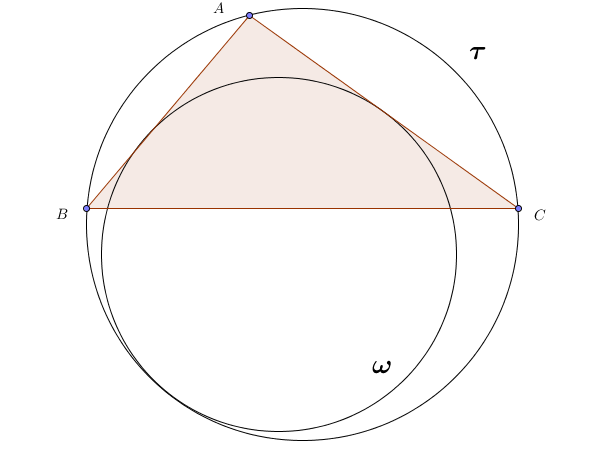# Incircle Frustation

Geometry Level 3Let $\Delta ABC$ be right-angled, with $\angle A = 90 ^ \circ$, & let its circumcircle be $\tau$. Let $\omega$ be the circle which touches sides $AB$ & $AC$ & circle $\tau$ (internally). Show that radius of $\omega$ is given by

$r = \frac{2bc}{a+b+c}$,

& find the ratio : $\frac {r}{r^c}$

where $r^c$ is the radius of the incircle of $\Delta ABC$.

Note : You can use the formula for $r$ directly, but prove it if you want an extra challenge!

×